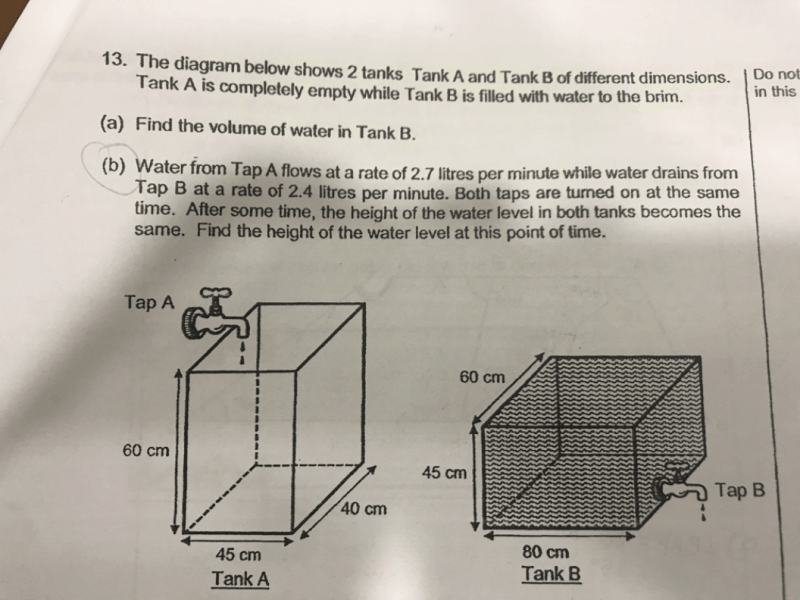# QuestionCan someone help me in this question ?? 🙏🏻

(a) Volume of water in Tank B → 80 × 60 × 45 = 216000 cm³

(b)

After the taps were turned on for t mins to reach height h,

Volume of water in Tank A → 40 × 45 × h = 2700t

1800h = 2700t

t = 1800h/2700 = 2h/3

Volume decreased in Tank B → 80 × 60 × (45 – h) = 2400t

216000 – 4800h = 2400t

216000 – 4800h = 2400 × 2h/3

216000 – 4800h = 1600h

6400h = 216000

h = 33.75 cm

0 Replies 0 Likes